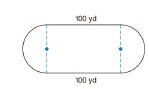Chapter 8.4, Problem 36E### Elementary Geometry for College St...

6th Edition
Daniel C. Alexander + 1 other
ISBN: 9781285195698

#### Solutions

Chapter
Section### Elementary Geometry for College St...

6th Edition
Daniel C. Alexander + 1 other
ISBN: 9781285195698
Textbook Problem
1 views

# In Exercises 34-44, use your calculator value of π unless otherwise stated. Round answers to two decimal places.A track is to be constructed around the football field at a high school. If the straightaways are 100 yd in length, what length of radius is needed for each of the semicircle shown if the total length around the track is to be 440 yd.To determine

To Find:

The length of the radius of the semi-circular pathway of the football field.

Explanation

Calculation:

A total length around the track is 440 yd.

The length of the straight ways are 100+100=200.

Therefore, the length of the straightaways is 200yd.

The length of the semi-circular pathways is 440200=220.

Therefore, the circumference of the circular pathway is 220 yd.

We know that the circumference of the circle is C=2πr

### Still sussing out bartleby?

Check out a sample textbook solution.

See a sample solution

#### The Solution to Your Study Problems

Bartleby provides explanations to thousands of textbook problems written by our experts, many with advanced degrees!

Get Started